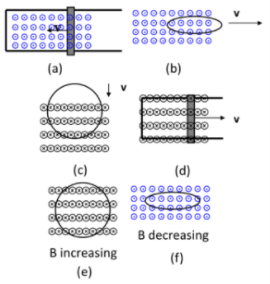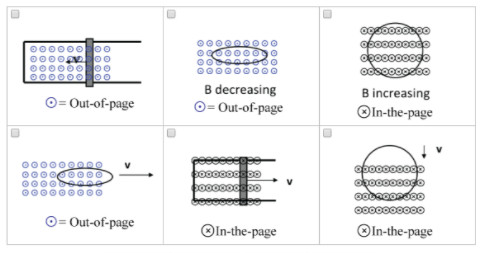# Problem: In the questions below there are 6 induction processes. The circle with the dot denotes a magnetic field pointing out of and the circle with the x denotes a magnetic field pointing into the screen. A line represents a conductor, while a bar denotes a sliding conductor. An arrow labeled "v" indicates the direction in which the conductor or sliding conductor is moving. Use Lenz's law to determine which of induced currents have a counterclockwise direction. Choose all that apply.

###### FREE Expert Solution

Lenz's law states that when an emf is generated by a change in the magnetic flux, according to Faraday's law, the polarity of the induced emf produces a current whose magnetic field is opposing the change that produced it.

Faraday's law mentioned, states that any change in the magnetic surrounding or a coil of wire causes an emf to be induced in the coil.

91% (414 ratings)###### Problem Details

In the questions below there are 6 induction processes. The circle with the dot denotes a magnetic field pointing out of and the circle with the x denotes a magnetic field pointing into the screen. A line represents a conductor, while a bar denotes a sliding conductor. An arrow labeled "v" indicates the direction in which the conductor or sliding conductor is moving.Use Lenz's law to determine which of induced currents have a counterclockwise direction. Choose all that apply.Frequently Asked Questions

What scientific concept do you need to know in order to solve this problem?

Our tutors have indicated that to solve this problem you will need to apply the Lenz's Law concept. You can view video lessons to learn Lenz's Law. Or if you need more Lenz's Law practice, you can also practice Lenz's Law practice problems.

What professor is this problem relevant for?

Based on our data, we think this problem is relevant for Professor Covell's class at UNCG.